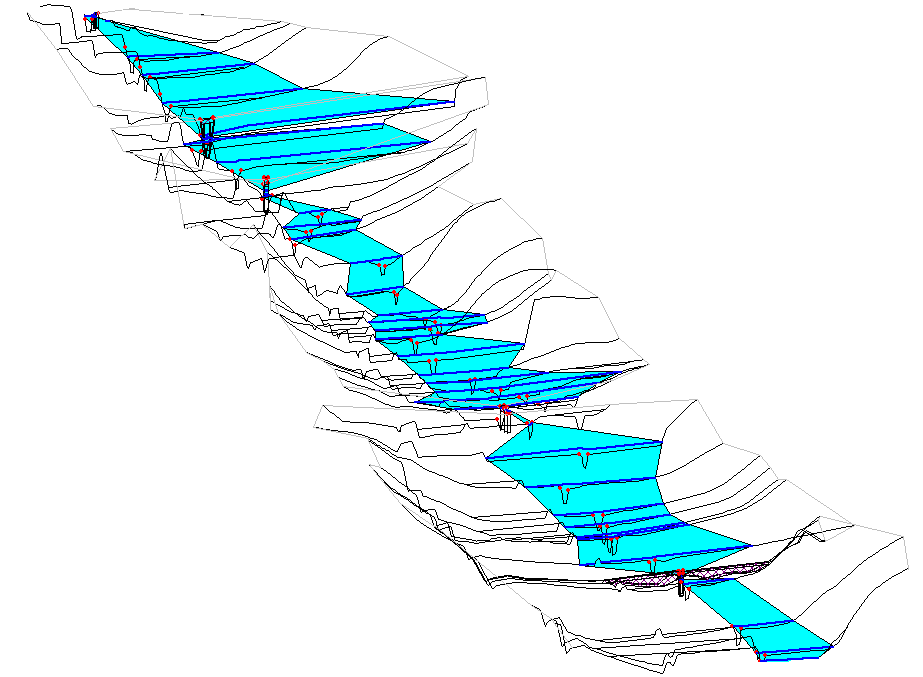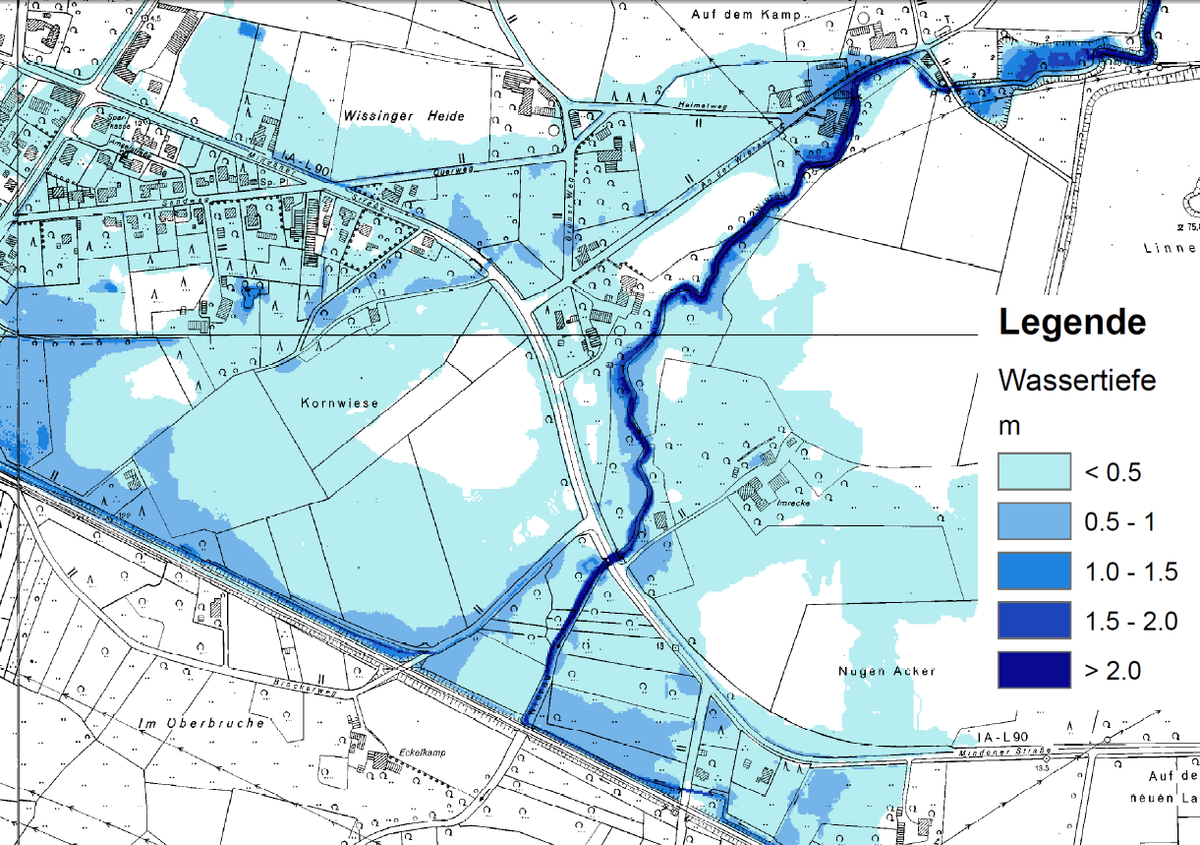# What are the basic calculations of hydraulic design

## Hydraulic calculation

A hydrodynamic-numerical model (HN model) required. A "hydrodynamic“The model is able to take into account the decisive forces such as gravity, frictional resistance and inertia, which are responsible for the flow processes. The solution of the complex mathematical process equations that describe the flow process in surface water is usually only possible through a "numerical“Discretization of the continuum (space and time) possible.Input and output data from HN models

Depending on the objective and the dimensional resolution of the quantities sought - above all water level and flow velocity - the basic equations can be subjected to various simplifications without significantly reducing their validity. This results in models with different advantages and disadvantages:

• 3-dimensional models: 3D HN models are used in small-scale calculation fields, in the flow around structures and in inlet structures of hydropower plants, where the mapping of possible eddies and the knowledge of all three velocity components is important. The great effort involved in the creation, together with the enormous need for computing capacity, remains the limiting factor. For this reason, 3D models are not used for flood modeling. The complete Navier-Stokes equations for the x, y and z directions are used to calculate the flow. Widely used 3D HN models include Flow 3D, MIKE 3D, ANSYS CFX, Fluent and Delft 3D.
• 2-dimensional models: With the 2D-HN models, one does without the information of a direction (mostly the vertical) and is content with its mean value instead. The equations averaged over the flow depth (the so-called "shallow water equations") enable an exact determination of the flow parameters (flow velocity v, water level WS, bed shear stress τ, ...). For rivers with flooded foreland and the formation of speed gradients across the direction of flow, the 2D HN models represent a good compromise between the creation effort and the accurate mapping of the flow processes. These models are commonly used successfully in the calculation of flood waves. The area of ​​application ranges from the investigation of local property protection to the determination of flood areas of several square kilometers. Common software packages for this area are InfoWorks ICM, Hydro_AS-2D, MIKE 21, SOBEK, FloodArea and Flow 2D.
• 1-dimensional models: The solution of 1D-HN models is several orders of magnitude faster than the 2D and 3D models. The distribution of the flow parameters (WS, v, ...) across the direction of flow is averaged in 1D models using the “Saint-Venant equations”. The parameters are therefore only calculated in the main flow direction. It may be possible to divide the calculation for the main bed or foreland / bank zones (bodies of water with structured cross-sections). The typical area of ​​application is the determination of the water level line in flowing waters with predominantly uniform cross-sections. The flow paths examined can range from a few hundred meters to a few hundred kilometers. There is a wide variety of 1D-HN models including: InfoWorks ICM, HEC-RAS, Jabron, WSPWIN, MIKE 11 and FLUSS.

Where there is a homogeneous foreland and the current runs parallel to the valley axis, a horizontal position of the water level perpendicular to the main direction of flow can be assumed. In this case, it is widespread practice to use 1D-HN models for the water level and then expand them orthogonally to the valley axis to the surrounding foreland. From the difference with the DTM and by means of interpolation, a 2D topic with the water depth (WT) can be created. The areas with WT> 0 represent the embankment. Some software provide corresponding GIS interfaces such as HEC-GeoRAS.Embankment from the intersection of the 1D-HN model HEC-RAS with the DGM

Hybrid models are being used more and more frequently, in which the 1D river sections are coupled with 2D areas where overflow is expected or the desired degree of detail is higher.

The choice of the right model is also dictated by the size of the area (limited river section, total catchment area) and the availability of basic data (digital terrain model DGM, terrestrial survey).

A clear advantage of a 2D HN model is that it can output the velocity vector for each element or each cell in which the area is subdivided. The 1D models differentiate at most between the speed in the river and on the foreland. The direction of flow is not calculated for this, but is given in the geometry.

Although all natural processes are time-dependent (unsteady), a flood wave may be slow enough to be passed through a sequence of time-independent (stationary) To be able to simulate states with sufficient accuracy.

With regard to the time dependency (e.g. of the discharge Q on the time t), a distinction is made between:

• Stationary modelsthat describe a state where the changes over time are negligible [Q = const],
• quasi-stationary modelsthat simulate the changes over time as a sequence of stationary states [Q = Q (t1), Q (t2) ... Q (tn)] and
• unsteady modelsthat preserve the time dependence of the processes [Q = Q (t)].

Only transient models can take into account the inherent flattening process of a flood wave (retention) as well as the effect of retention areas. Although the wave damping in quasi-stationary models can be taken into account by an upstream hydrological model, only the transient models guarantee a correct volume balance (compliance with the continuity condition). Stationary models, on the other hand, are not true to volume.

The transient approach when calculating floods is always preferable if the inflows are available or estimated as a hydrograph Q = Q (t).

One should take into account that the computational effort increases disproportionately with the dimensionality, the unsteadiness and the expansion of the model.

### Model creation

In order to set up an HN model to simulate a flood, various basic data are required:

• inflow from the upper reaches, discharges from the sewer network and side inflows: As a hydrograph Q (t) for a transient calculation or as constant values ​​for the quasi-stationary and the stationary approach (upper or internal boundary condition).
• Speed ​​distribution at the inflow edge for 2D and 3D models.
• Water level (1D) or water level distribution (2D and 3D) or runoff-water level relationship (key curve) at the outlet from the model (lower boundary condition).
• topography of the river bed and the foreland as a digital terrain model (DGM) or terrestrial survey, LiDAR or LaserScan data.
• Suitable Roughness coefficients for the different properties of the bed, embankments, structures, vegetation and foreland. A water inspection by competent specialists is essential for this.
• Initial conditions: For the unsteady models, the initial value of the decisive parameters (water level and speed vector) must also be specified in the entire model area.

Both in 1D and 2D HN models, the existing transverse structures (bridges, culverts, weirs, bed sills, ...) and other structures that serve to control the runoff (weirs, regulating bodies and, in the case of 1D, also polders) must be maintained manually.Floods as a result of an HQ100 at the Wierau (2D HN model FloodArea)

### Calibration and validation

Despite their conceptual regularity, the flow equations also contain empirical approaches (friction losses, fouling effects, turbulence models, ...). In the case of HN models, the roughness is usually the parameter afflicted with the greatest uncertainty. Every model therefore absolutely needs one calibration: The empirical parameters are varied in an iterative process until the calculated results (mostly the water level) show a sufficiently good agreement with the directly measured or indirectly derived values. After a flood, for example, the so-called flood line (deposit of floating debris at the highest water level) is usually easily recognizable. Often the moisture on facades or a certain amount of abrasion on bridge piers is visible for a long time. There may be high water marks for historical events. If available, level data can of course also be used for calibration.

With the calibration, the model is adjusted in the event of an event so that the results agree with the observation. The check of whether the selected parameter set also retains its validity for other events is called Validation. It verifies the model quality, i.e. the transferability of the model to events other than the calibration (e.g. floods with different return times). Different parameter sets may have to be selected.

< back